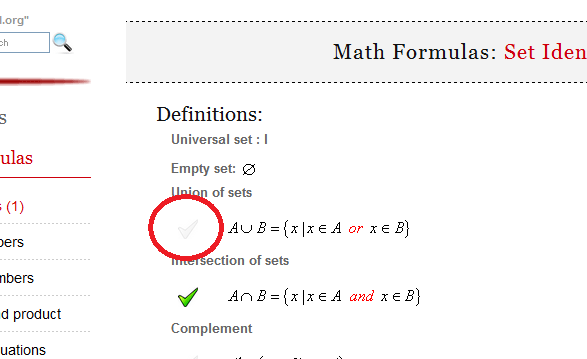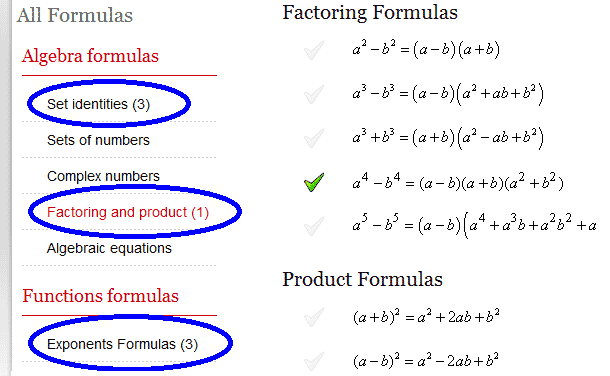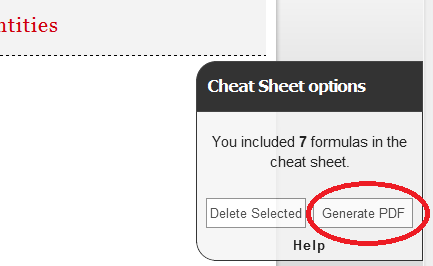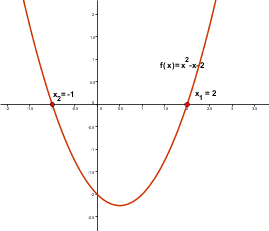Math Calculators, Lessons and Formulas

It is time to solve your math problem

mathportal.org

You included 0 formulas in your custom cheat sheet.

Cheat Sheet options

How to generate cheat sheet ?

To create cheat sheet first you need to select formulas which you want to include in it. To select formula click at picture next to formula.You can choose formulas from different pages.After you have selected all the formulas which you would like to include in cheat sheet, click the "Generate PDF" button.Math Formulas: Solutions of algebraic equations

Quadric Equation: $ax^2 + bx + c = 0$Solutions (roots):

 $$x_{1,2} = \frac{-b \pm \sqrt{b^2 - 4ac}}{2a}$$

If $D = b^2 - 4ac$ is the discriminant , then the roots are

1. real and unique if $D > 0$

2. real and equal if $D = 0$

3. complex conjugate if $D < 0$

Cubic Equation: $x^3 + a_1x^2 + a_2x + a_3 = 0$

Let

 \begin{aligned} Q &= \frac{3a_2 - a_1^2}{9} \\ R &= \frac{9a_1a_2 - 27a_3 - 2a_1^3}{54} \\ S &= \sqrt[\Large3]{R + \sqrt{Q^3 + R^2}} \\ T &= \sqrt[\Large3]{R - \sqrt{Q^3 + R^2}} \end{aligned}

Then solutions (roots) of the cubic equation are:

 \begin{aligned} x_1 &= S + T - \frac{1}{3}a_1 \\ x_2 &= -\frac{1}{2} (S + T) - \frac{1}{3}a_1 + \frac{1}{2}\,i\,\sqrt{3}(S-T) \\ x_3 &= -\frac{1}{2} (S + T) - \frac{1}{3}a_1 - \frac{1}{2}\,i\,\sqrt{3}(S-T) \end{aligned}

If $D = Q^3 + R^2$ is the discriminant of the cubic equation, then:

1. one root is real and two complex conjugate if $D > 0$

2. all roots are real and at last two are equal if $D=0$

3. all roots are real and unequal if $D < 0$

Quartic Equation:$x^4 + a_1x^3 + a_2x^2 + a_3x + a_4 = 0$

Let $y_1$ be a real root of the cubic equation

 $$y^3 - a_2y^2 + (a_1a_3-4a_4)y+(4a_2a_4 - a_3^2 - a_1^2a_4) = 0$$

Then solutions of the quartic equation are the 4 roots of

 $$z^2 + \frac{1}{2}\left(a_1 \pm \sqrt{a_1^2 - 4a_2+4y_1}\right)z + \frac{1}{2}\left(y_1 \pm \sqrt{y_1^2 - 4a_4}\right)= 0$$

Were these formulas helpful?

Yes No